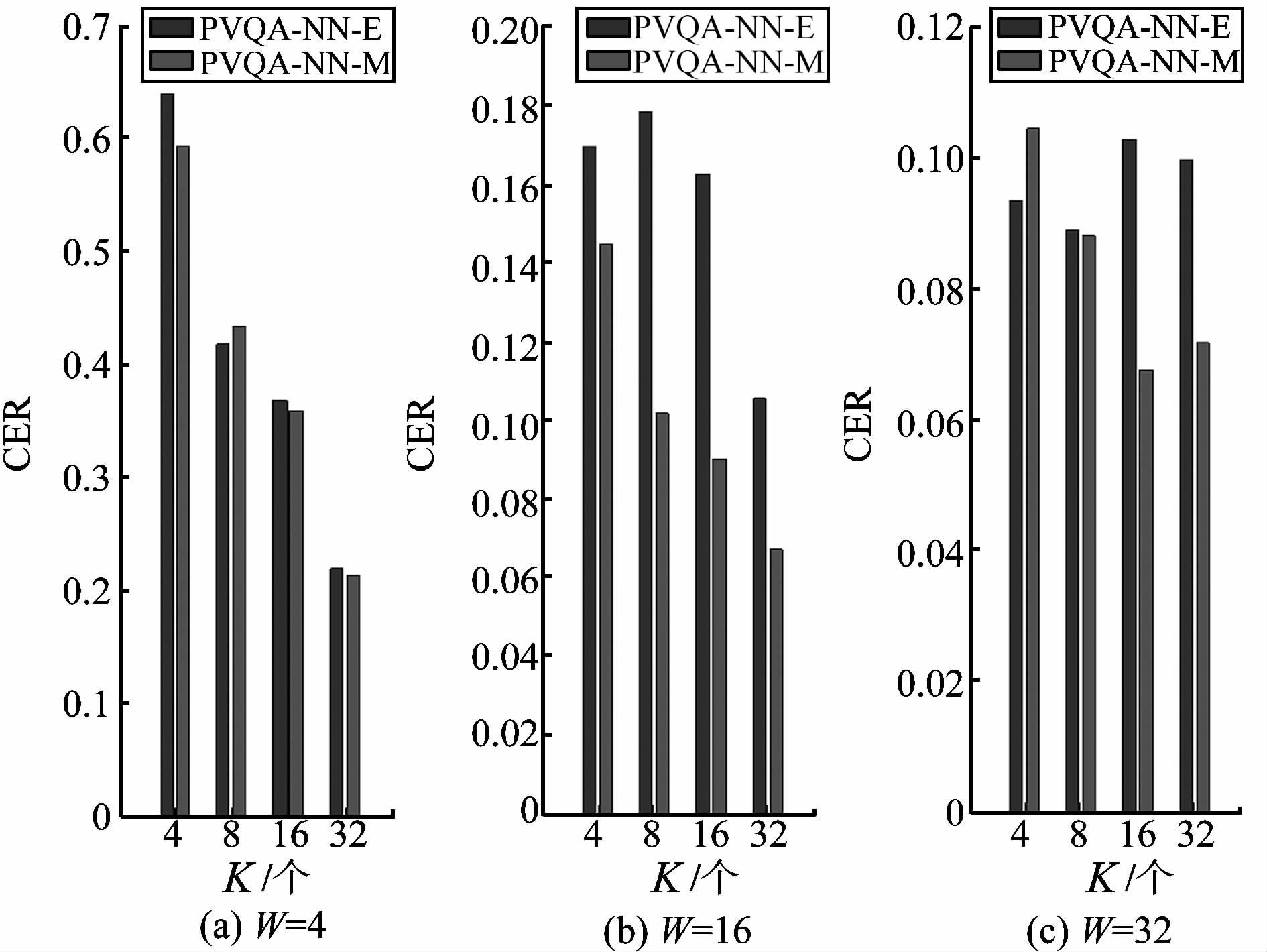﻿ 基于马氏距离的分段矢量量化时间序列分类文章快速检索 高级检索
 山东大学学报(工学版)2016, Vol. 46Issue (3): 51-57  DOI: 10.6040/j.issn.1672-3961.2.2015.0500

### 引用本文TAO Zhiwei, ZHANG Li. Time series classification using piecewise vector quantized approximation based on Mahalanobis distance[J]. Journal of Shandong University(Engineering Science), 2016, 46(3): 51-57. DOI: 10.6040/j.issn.1672-3961.2.2015.050.### 文章历史

1. 苏州大学计算机科学与技术学院, 江苏 苏州 215006;
2. 江苏省计算机信息处理技术重点实验室, 江苏 苏州 215006

Time series classification using piecewise vector quantized approximation based on Mahalanobis distance
TAO Zhiwei1, ZHANG Li1,21. School of Computer Science and Technology, Soochow University, Suzhou 215006, Jiangsu, China ;
2. Provincial Key Laboratory for Computer Information Processing Technology, Soochow University, Suzhou 215006, Jiangsu, China
Abstract: A Mahalanobis distance-based time series classification using PVQA (MPVQA) algorithm was developed. On the basis of inheriting the time complexity of the traditional algorithm and by exploiting Mahalanobis distance, the algorithm could easily overcome the default that the Euclidean distance was easily influenced by the mode characteristic dimension and improve the accuracy. PVQA was first used to generate a codebook using training samples, and then the Mahalanobis distance was taken as the measure of similarity and used to reconstruct time subsequences. For an unseen time series, the Mahalanobis distance was also adopted to find the most similar one to it. Experimental results on four time series datasets demonstrated that our method was more powerful to classify the time series.
Key words: Mahalanobis distance    piecewise vector quantized approximation    time series    codebook    Euclidean distance    characteristic dimension    reconstruct
0 引言

PVQA对于时间序列有较好的降维效果[8-9],本文采用PVQA作为时间序列进行降维。PVQA对时间序列降维分为两步:先把时间序列分割成等长的时间子序列,并根据这些时间子序列生成相应的码本;然后使用码词替代给定的训练时间子序,并重构时间序列,达到降维的目的。若要对未知时间序列进行处理,则用同样的方法重构该时间序列,然后采用最近邻策略,计算未知重构样例与训练重构样例的最小欧氏距离,从而判断未知样例的类别。在PVQA的重构过程中也采用了欧氏距离作为相似性度量。由于欧氏距离受模式特征量纲的影响,不一定是最好的相似性度量选择。其他常见的相似性度量还有余弦相似度(cosine similarity,CS)、马氏距离(Mahalanobis distance,MD)等。

1 矢量量化

 $minf\left( X,K \right)=\frac{1}{mn}\sum\limits_{i=1}^{n}{\|{{x}_{i}}-g\left( {{x}_{i}} \right){{\|}^{2}}},$

2 基于马氏距离的分段矢量量化

2.1 马氏距离图 1 基于马氏距离的分段矢量量化分类算法框架 Figure 1 The framework of MPVQA

 ${{d}_{M}}\left( x,y \right)=\sqrt{\|x-y\|_{M}^{2}}\text{ }=\sqrt{\left( x-y \right)P{{\left( x-y \right)}^{T}}}。$ (1)
2.2 码本生成

2.3 基于马氏距离的重构

 ${{p}_{j}}=\underset{k=1,2,\ldots ,K}{\mathop{arg\text{ }min}}\,\text{ }{{d}_{M}}\left( {{x}^{j}},{{c}_{k}} \right),$

cpj与xj最为相似。此时,式(1)中的矩阵P 为码本的协方差矩阵。

2.4 基于马氏距离的分类

 ${{d}_{M}}\left( {{c}_{i}},{{c}_{j}} \right)=\sqrt{\left( {{c}_{i}}-{{c}_{j}} \right)C{{\left( {{c}_{i}}-{{c}_{j}} \right)}^{T}}},$

 $A=\left[ \begin{matrix} {{a}_{11}} & {{a}_{12}} & \cdots & {{a}_{1k}} \\ {{a}_{21}} & {{a}_{22}} & \cdots & {{a}_{2k}} \\ \vdots & \vdots & \ddots & \vdots \\ \text{ }{{a}_{k1}} & {{a}_{k2}} & \cdots & {{a}_{kk}} \\ \end{matrix} \right]\text{ },$

3 仿真试验

3.1 Control数据集

3.1.1 码本生成图 2 Control数据集中分段数为10大小为16的码本 Figure 2 The codebook of size 16 when segments is 10 in the control dataset
3.1.2 重构和分类图 3 原始测试时间序列以及重构测试时间序列 Figure 3 The original test time series and the reconstructed time series表 1 测试样本与其近邻的距离 Table 1 The distance between test sample and its nearest neighbor图 4 训练时间序列x′和x″的重构 Figure 4 The reconstructed training time series x′ and x″
3.1.3 分类结果

 $CER=\frac{{{n}_{w}}}{{{n}_{t}}}$

3.2 50Words数据集图 5 Control数据集上分类错误率对比 Figure 5 The comparison of the classification error in control dataset
3.3 Two Patterns数据集图 6 50Words数据集上分类错误率对比 Figure 6 The comparisonof classification error in 50Words dataset表 2 50Words数据集上的最优分类效果比较 Table 2 The comparison of optimal classification effect in 50Words dataset图 7 Two Patterns数据集上分类错误率对比 Figure 7 The classification error comparison in Two Patternsdataset
3.4 Swedishleaf数据集表 3 Two Patterns数据集上的最优分类效果比较 Table 3 The comparison of optimal classification effect in Two Patterns dataset图 8 Swedishleaf数据集上分类错误率对比 Figure 8 The comparisonof classification error in Swedishleafdataset表 4 Swedishleaf数据集上的最优分类效果比较 Table 4 The comparison of optimal classification effect in Swedishleaf dataset
4 结语

  FALOUTSOS C, RANGANATHAN M, MANOLOPOULOS Y, et al. Fast subsequence matching in time-series databases[J]. ACM Sigmod Record,2000, 23 (2) : 419-429. (0)  CHAN K P, FU A W C. Efficient time series matching by wavelets[C]//Proceedings of IEEE International Conference on Data Engineering. Sydney, NSW:IEEE, 1999:126-133. (0)  CHAN F K P, FU A W, YU C. Haar wavelets for efficient similarity search of time-series: with and without time warping[J]. Knowledge and Data Engineering, IEEE Transactions on,2003, 15 (3) : 686-705. (0)  LIN J, KEOGH E, WEI L, et al. Experiencing SAX: a novel symbolic representation of time series[J]. Data Mining and knowledge discovery,2007, 15 (2) : 107-144. (0)  LI H, YANG L. Time series visualization based on shape features[J]. Knowledge-Based Systems,2013, 41 (1) : 43-53. (0)  KEOGH E, LIN J, FU A. Hot sax: efficiently finding the most unusual time series subsequence[J]. Data Mining,2005, 11 (1) : 226-233. (0)  DELATHAUWER L, DE Moor B, VANDEWALLE J. A multilinear singular value decomposition[J]. SIAM journal on Matrix Analysis and Applications,2000, 21 (4) : 1253-1278. (0)  WANG Q, MEGALOOIKONOMOU V. A dimensionality reduction technique for efficient time series similarity analysis[J]. Information Systems,2008, 33 (1) : 115-132. (0)  LI H, YAND L, GUO C. Improved piecewise vector quantized approximation based on normalized time subsequences[J]. Measurement,2013, 46 (9) : 3429-3439. (0)  DING H, TRAJCEVSKI G, SCHEUERMANN P, et al. Querying and mining of time series data: experimental comparison of representations and distance measures[J]. Proceedings of the VLDB Endowment,2008, 1 (2) : 1542-1552. (0)  DONG Y, SUN Z, JIA H. A cosine similarity-based negative selection algorithm for time series novelty detection[J]. Mechanical Systems and Signal Processing,2006, 20 (6) : 1461-1472. (0)  DEMAESSCHALCK R, JOUAN-RIMBAUD D, MASSART D L. The Mahalanobis distance[J]. Chemometrics and Intelligent Laboratory Systems,2000, 50 (1) : 1-18. (0)  WANG X J, WANG L. Applications of Topsis improved based on Mahalanobis distance in supplier selection[J]. Control and Decision,2012, 27 (10) : 1566-1570. (0)  ZHU W D, HU J L. Sparse representation classification algorithm based on Mahalanobis distance[J]. Computer Technology and Development,2011, 21 (11) : 27-30. (0)  SASIKALA I S, BANU N. Privacy Preserving data mining using piecewise vector quantization (PVQ)[J]. International Journal of Advanced Research in Computer Science ＆ Technology,2014, 2 (3) : 302-306. (0)  LU Z M, WANG J X, LIU B B. An improved lossless data hiding scheme based on image VQ-index residual value coding[J]. Journal of Systems and Software,2009, 82 (6) : 1016-1024. (0)  WANG Q, MEGALOOIKONOMOU V, FALOUTSOS C. Time series analysis with multiple resolutions[J]. Information Systems,2010, 35 (1) : 56-74. (0)  HUANG F, ZHOU J, LU X D. The simulation of one-dimensional range profile recognition based on Mahalanobisdistance[J]. Computer Simulation,2010, 27 (3) : 31-34. (0)  MEGALOOIKONOMOU V, LI G, WANG Q. A dimensionality reduction technique for efficient similarity analysis of time series databases[C]//Proceedings of the thirteenth ACM international conference on Information and knowledge management. New York, USA:ACM, 2004:160-161. (0)  KEOGH E. Welcome to the UCR time series classification/clustering page [DB/OL]. [2015-03-12]. http://www.cs.ucr.edu/～eamonn/time-series-data/. (0)